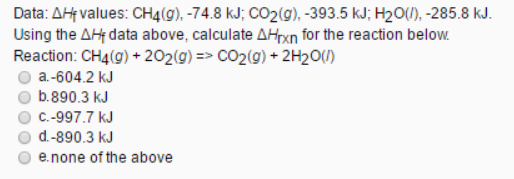# Problem: Data: ΔHf values: CH4 (g), -74.8 kJ: CO2 (g), -393.5 kJ: H2O (l), -285.8 kJ. Using the ΔHf data above, calculate ΔHrxn for the reaction below. Reaction: CH4 (g) + 2O2 (g) → CO2 (g) + 2H2O (l) a. -604.2 kJ b. 8.90.3 kJ c. -997.7 kJ d. -890.3 kJ e. none of the above

###### FREE Expert Solution
92% (52 ratings)###### Problem Details

Data: ΔHf values: CH4 (g), -74.8 kJ: CO2 (g), -393.5 kJ: H2O (l), -285.8 kJ.

Using the ΔHf data above, calculate ΔHrxn for the reaction below.

Reaction: CH4 (g) + 2O2 (g) → CO2 (g) + 2H2O (l)

a. -604.2 kJ

b. 8.90.3 kJ

c. -997.7 kJ

d. -890.3 kJ

e. none of the above